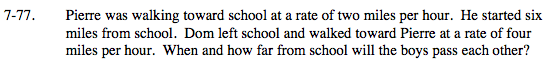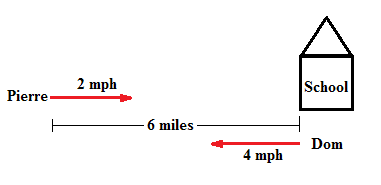### Home > MC2 > Chapter 7 > Lesson 7.2.2 > Problem7-77

7-77.Drawing a picture may be helpful.

Try and write an expression to represent these actions.

Notice that Pierre and Dom must walk a combined total of 6 miles.To find the amount of miles one person walks, you must multiply the rate by the time.
Let x represent the amount of time the two will have walked when they pass each other.

Since the two must walk a total of 6 miles:

At 1 hour, how many miles will Pierre have walked?
How far will he be from school?

2x + 4x = 6
Solve.

x = 1 hour